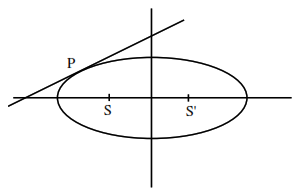# On the ellipse

Question:

On the ellipse $\frac{x^{2}}{8}+\frac{y^{2}}{4}=1$ let $P$ be a point in the second quadrant such that the tangent at $P$ to the ellipse is perpendicular to the line $x+2 y=0 .$ Let $S$ and $S^{\prime}$ be the foci of the ellipse and e be its eccentricity. If $\mathrm{A}$ is the area of the triangle $\mathrm{SPS}^{\prime}$ then, the value of $\left(5-\mathrm{e}^{2}\right) . \mathrm{A}$ is :

1. 6

2. 12

3. 14

4. 24

Correct Option: 1

Solution:Equation of tangent: $y=2 x+6$ at $\mathrm{P}$

$\therefore \mathrm{P}(-8 / 3,2 / 3)$

$e=\frac{1}{\sqrt{2}}$

$S \& S^{\prime}=(-2,0) \&(2,0)$

Area of $\Delta \mathrm{SPS}^{\prime}=\frac{1}{2} \times 4 \times \frac{2}{3}$

$A=\frac{4}{3}$

$\therefore\left(5-\mathrm{e}^{2}\right) \mathrm{A}=\left(5-\frac{1}{2}\right) \frac{4}{3}=6$